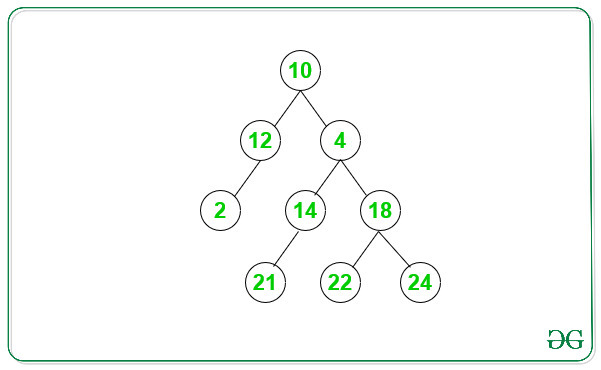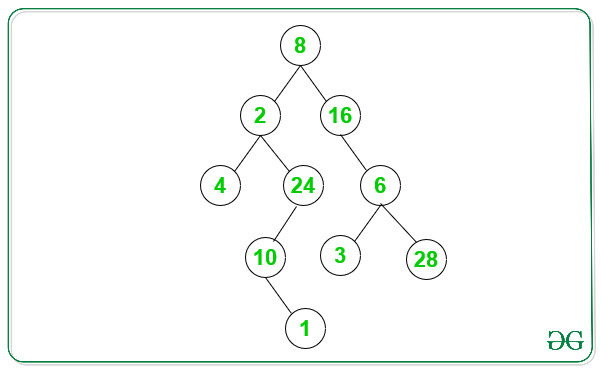# Count even paths in Binary Tree

Given a Binary Tree, the task is to count the number of even paths in the given Binary Tree. Even Path is a path where root to leaf path contains all even nodes only.

Examples:

Input: Below is the given Binary Tree:Output: 3
Explanation:
There are 3 even path for the above Binary Tree:
1. 10->12->2
2. 10->4->18->22
3. 10->4->18->24

Input: Below is the given Binary Tree:Output: 2
Explanation:
There are 2 even path for the above Binary Tree:
1. 8->2->4
2. 8->16->6->28

## Recommended: Please try your approach on {IDE} first, before moving on to the solution.

Naive Approach: The idea is to generate all the root to leaf path and check whether all nodes in every path is even or not. Count all the paths with even nodes in it and return the count. The above implementation takes extra space to store the path.

Efficient Approach: The idea is to use Preorder Tree Traversal. During preorder traversal of the given binary tree do the following:

1. If current value of the node is odd or pointer becomes NULL then return the count.
2. If the current node is a leaf node then increment the count by 1.
3. Recursively call for the left and right subtree with the updated count.
4. After all recursive call, the value of count is number of even paths for a given binary tree.

Below is the implementation of the above approach:

## C++

 `// C++ program for the above approach ` `#include ` `using` `namespace` `std; ` ` `  `// A Tree node ` `struct` `Node { ` `    ``int` `key; ` `    ``struct` `Node *left, *right; ` `}; ` ` `  `// Utility function to create a new node ` `Node* newNode(``int` `key) ` `{ ` `    ``Node* temp = ``new` `Node; ` `    ``temp->key = key; ` `    ``temp->left = temp->right = NULL; ` `    ``return` `(temp); ` `} ` ` `  `// Utility function to count the even path ` `// in a given Binary tree ` `int` `evenPaths(``struct` `Node* node, ``int` `count) ` `{ ` ` `  `    ``// Base Condition, when node pointer ` `    ``// becomes null or node value is odd ` `    ``if` `(node == NULL || (node->key % 2 != 0)) { ` `        ``return` `count; ` `    ``} ` ` `  `    ``// Increment count when encounter leaf ` `    ``// node with all node value even ` `    ``if` `(!node->left && !node->right) { ` `        ``count++; ` `    ``} ` ` `  `    ``// Left recursive call, and save the ` `    ``// value of count ` `    ``count = evenPaths(node->left, count); ` ` `  `    ``// Right reursive call, and return ` `    ``// value of count ` `    ``return` `evenPaths(node->right, count); ` `} ` ` `  `// Function to count the even paths in a ` `// given Binary tree ` `int` `countEvenPaths(``struct` `Node* node) ` `{ ` ` `  `    ``// Function call with count = 0 ` `    ``return` `evenPaths(node, 0); ` `} ` ` `  `// Driver Code ` `int` `main() ` `{ ` ` `  `    ``// Tree ` `    ``Node* root = newNode(12); ` `    ``root->left = newNode(13); ` `    ``root->right = newNode(12); ` ` `  `    ``root->right->left = newNode(14); ` `    ``root->right->right = newNode(16); ` ` `  `    ``root->right->left->left = newNode(21); ` `    ``root->right->left->right = newNode(22); ` `    ``root->right->right->left = newNode(22); ` `    ``root->right->right->right = newNode(24); ` `    ``root->right->right->right->left = newNode(8); ` ` `  `    ``// Function call ` `    ``cout << countEvenPaths(root); ` `    ``return` `0; ` `} `

## Java

 `// Java program for the above approach ` `import` `java.util.*; ` `class` `GFG{ ` ` `  `    ``// A Tree node ` `    ``static` `class` `Node { ` `        ``int` `key; ` `        ``Node left, right; ` `    ``}; ` `     `  `    ``// Utility function to create a new node ` `    ``static` `Node newNode(``int` `key) ` `    ``{ ` `        ``Node temp = ``new` `Node(); ` `        ``temp.key = key; ` `        ``temp.left = temp.right = ``null``; ` `        ``return` `(temp); ` `    ``} ` `     `  `    ``// Utility function to count the even path ` `    ``// in a given Binary tree ` `    ``static` `int` `evenPaths(Node node, ``int` `count) ` `    ``{ ` `     `  `        ``// Base Condition, when node pointer ` `        ``// becomes null or node value is odd ` `        ``if` `(node == ``null` `|| (node.key % ``2` `!= ``0``)) { ` `            ``return` `count; ` `        ``} ` `     `  `        ``// Increment count when encounter leaf ` `        ``// node with all node value even ` `        ``if` `(node.left == ``null` `&& node.right == ``null``) { ` `            ``count++; ` `        ``} ` `     `  `        ``// Left recursive call, and save the ` `        ``// value of count ` `        ``count = evenPaths(node.left, count); ` `     `  `        ``// Right reursive call, and return ` `        ``// value of count ` `        ``return` `evenPaths(node.right, count); ` `    ``} ` `     `  `    ``// Function to count the even paths in a ` `    ``// given Binary tree ` `    ``static` `int` `countEvenPaths(Node node) ` `    ``{ ` `     `  `        ``// Function call with count = 0 ` `        ``return` `evenPaths(node, ``0``); ` `    ``} ` `     `  `    ``// Driver Code ` `    ``public` `static` `void` `main(String args[]) ` `    ``{ ` `     `  `        ``// Tree ` `        ``Node root = newNode(``12``); ` `        ``root.left = newNode(``13``); ` `        ``root.right = newNode(``12``); ` `     `  `        ``root.right.left = newNode(``14``); ` `        ``root.right.right = newNode(``16``); ` `     `  `        ``root.right.left.left = newNode(``21``); ` `        ``root.right.left.right = newNode(``22``); ` `        ``root.right.right.left = newNode(``22``); ` `        ``root.right.right.right = newNode(``24``); ` `        ``root.right.right.right.left = newNode(``8``); ` `     `  `        ``// Function call ` `        ``System.out.println(countEvenPaths(root)); ` `         `  `    ``} ` `} ` ` `  `// This code is contributed by AbhiThakur `

## C#

 `// C# program for the above approach ` `using` `System; ` ` `  `class` `GFG{ ` `  `  `    ``// A Tree node ` `    ``class` `Node { ` `        ``public` `int` `key; ` `        ``public` `Node left, right; ` `    ``}; ` `      `  `    ``// Utility function to create a new node ` `    ``static` `Node newNode(``int` `key) ` `    ``{ ` `        ``Node temp = ``new` `Node(); ` `        ``temp.key = key; ` `        ``temp.left = temp.right = ``null``; ` `        ``return` `(temp); ` `    ``} ` `      `  `    ``// Utility function to count the even path ` `    ``// in a given Binary tree ` `    ``static` `int` `evenPaths(Node node, ``int` `count) ` `    ``{ ` `      `  `        ``// Base Condition, when node pointer ` `        ``// becomes null or node value is odd ` `        ``if` `(node == ``null` `|| (node.key % 2 != 0)) { ` `            ``return` `count; ` `        ``} ` `      `  `        ``// Increment count when encounter leaf ` `        ``// node with all node value even ` `        ``if` `(node.left == ``null` `&& node.right == ``null``) { ` `            ``count++; ` `        ``} ` `      `  `        ``// Left recursive call, and save the ` `        ``// value of count ` `        ``count = evenPaths(node.left, count); ` `      `  `        ``// Right reursive call, and return ` `        ``// value of count ` `        ``return` `evenPaths(node.right, count); ` `    ``} ` `      `  `    ``// Function to count the even paths in a ` `    ``// given Binary tree ` `    ``static` `int` `countEvenPaths(Node node) ` `    ``{ ` `      `  `        ``// Function call with count = 0 ` `        ``return` `evenPaths(node, 0); ` `    ``} ` `      `  `    ``// Driver Code ` `    ``public` `static` `void` `Main(String []args) ` `    ``{ ` `      `  `        ``// Tree ` `        ``Node root = newNode(12); ` `        ``root.left = newNode(13); ` `        ``root.right = newNode(12); ` `      `  `        ``root.right.left = newNode(14); ` `        ``root.right.right = newNode(16); ` `      `  `        ``root.right.left.left = newNode(21); ` `        ``root.right.left.right = newNode(22); ` `        ``root.right.right.left = newNode(22); ` `        ``root.right.right.right = newNode(24); ` `        ``root.right.right.right.left = newNode(8); ` `      `  `        ``// Function call ` `        ``Console.WriteLine(countEvenPaths(root)); ` `          `  `    ``} ` `} ` ` `  `// This code is contributed by PrinciRaj1992 `

Output:

```3
```

Time Complexity: O(N), where N is the number of nodes in the given binary tree.

Don’t stop now and take your learning to the next level. Learn all the important concepts of Data Structures and Algorithms with the help of the most trusted course: DSA Self Paced. Become industry ready at a student-friendly price.

My Personal Notes arrow_drop_upCheck out this Author's contributed articles.

If you like GeeksforGeeks and would like to contribute, you can also write an article using contribute.geeksforgeeks.org or mail your article to contribute@geeksforgeeks.org. See your article appearing on the GeeksforGeeks main page and help other Geeks.

Please Improve this article if you find anything incorrect by clicking on the "Improve Article" button below.